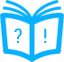# BioStat

BioStat is affordable and easy-to-use statistical analysis package dedicated to the needs of professionals working in the fields of medicine, biology, pharmacy and biochemistry. BioStat is based on the well-known StatPlus package and contains virtually all of its functionality, including ANOVA, regression and survival analysis. Thanks to familiar Excel-like look and feel and compatibility with major data formats one can benefit from a short learning curve.Standalone version reads numerous text formats, Microsoft* Excel* 97-2003 (XLS) and 2007-2021 (XLSX) workbooks, SPSS* Documents and supports almost all Excel built-in worksheet functions (math, statistical, financial). New version comes with Excel add-in with all the BioStat features available.

### Statistics

BioStat allows to perform various types of analysis - basic statistics and tables, ANOVA, regression analysis, non-parametric statistics, survival and power analysis. Analysis results are written into new worksheet and could be easily edited or exported.

View Features List

### Looking For More?

StatPlus includes all the BioStat features and even more: design of experiment, time series analysis and forecasting, control charts for quality control. StatPlus comes as both standalone spreadsheet and Excel add-in, and works on Windows and Mac OS.

### Free Trial

We have free trial that gives you an opportunity to evaluate the software before you purchase it. Should you have any questions during the trial period, please feel free to contact our Support Team.

### Affordable

You will benefit from the reduced learning curve and attractive pricing while enjoying the benefits of precise routines and calculations. License is permanent, there is no renewal charges.

Get a Quote Today

### Requirements

BioStat requires Windows 2000 or newer, Windows 8 or newer recommended. Excel add-in requires Excel 2007 or newer. BioStat supports Windows 11 and Excel 2021### Features List

• Pro Features
• Fast and powerful standalone spreadsheet.
• Add-in for Excel 2007, 2010, 2013, 2016, 2019 and 2021.
• Priority support.
• Options to emulate Excel Analysis ToolPak results and migration guide for users switching from Analysis ToolPak.
• Basic Statistics
• Detailed descriptive statistics.
• One-sample t-test.
• Two-sample t-test.
• Two-sample t-test for summarized data.
• Fisher F-test.
• One-sample and two-sample z-tests.
• Correlation analysis and covariance.
• Normality tests (Jarque-Bera, Shapiro-Wilk, Shapiro-Francia, Cramer-von Mises, Anderson-Darling, Kolmogorov-Smirnov, D'Agostino's tests).
• Cross-tabulation and Chi-square.
• Frequency tables analysis (for discrete and continuous variables).
• Multiple definitions for computing quantile statistics.
• Analysis of Variance (ANOVA)
• One-way and two-way ANOVA (with and without replications).
• Three-way analysis of variance.
• Post-hoc comparisons - Bonferroni, Tukey-Kramer, Tukey B, Tukey HSD, Neuman-Keuls, Dunnett.
• General Linear Models (GLM) ANOVA.
• Within subjects ANOVA and mixed models.
• Multivariate Analysis
• Principal component analysis (PCA).
• Factor analysis (FA).
• Discriminant function analysis.
• Hierarchical Clustering and K-Means.
• Nonparametric Statistics
• 2x2 tables analysis (Chi-square, Yates Chi-square, Exact Fisher Test, etc.).
• Rank and percentile.
• Chi-square test.
• Rank correlations (Kendall Tau, Spearman R, Gamma, Fechner).
• Comparing independent samples
Mann-Whitney U Test, Kolmogorov-Smirnov test, Wald-Wolfowitz Runs Test, Rosenbaum Criterion. Kruskal-Wallis ANOVA and Median test.
• Comparing dependent samples
Wilcoxon Matched Pairs Test, Sign Test, Friedman ANOVA, Kendall's W (coefficient of concordance).
• Cochran's Q Test.
• Regression Analysis
• Multivariate linear regression (residuals analysis, collinearity diagnostics, confidence and prediction bands).
• Weighted least squares (WLS) regression.
• Logistic regression.
• Stepwise (forward and backward) regression.
• Polynomial regression.
• Curve fitting.
• Tests for heteroscedasticity: Breusch–Pagan test (BPG), Harvey test, Glejser test, Engle's ARCH test (Lagrange multiplier) and White test.
• Survival Analysis
• Life tables.
• Kaplan-Meier (log rank test, hazard ratios).
• Cox proportional-hazards regression.
• Probit-analysis (Finney and LPM).
LD values (LD50/ED50 and others), cumulative coefficient calculation.
• Receiver operating characteristic curves analysis (ROC analysis).
AUC methods - DeLong's, Hanley and McNeil's. Report includes: AUC (with confidence intervals), curve coordinates, performance indicators - sensitivity and specificity (with confidence intervals), accuracy, positive and negative predictive values, Youden's J (Youden's index), Precision-Recall plot.
• Comparing ROC curves.
• Data Processing
• Sampling (random, periodic, conditional).
• Random numbers generation.
• Standardization.
• Stack/unstack operations.
• Matrix operations.
• Statistical Charts
• Histogram
• Scatterplot.
• Box plot.
• Stem-and-leaf plot.
• Bland-Altman plot.
• Bland-Altman plot with multiple measurements per subject.
• Quantile-quantile Q-Q plots for different distributions.
• Upgrade to StatPlus to get more:
• Time Series Analysis
• Fourier analysis.
• Interrupted time series analysis.
• Smoothing.
• Moving average.
• Autocorrelation (ACF and PACF).
• Unit root tests - Dickey–Fuller, Augmented Dickey–Fuller (ADF), Phillips–Perron, Kwiatkowski–Phillips–Schmidt–Shin (KPSS).
• Control charts - X-bar, R-chart, S-chart, I-MR chart, P-chart, C-chart, U-chart, CUSUM-chart.
• Multi-platform
• StatPlus is available for PC, Mac and iOS.